# S.I. No. 185/2008 - Asset Covered Securities Act 2001 (Sections 91(1) and 41B) (Sensitivity to Interest Rate Changes Commercial Mortgage Credit) Regulations 2008

 Notice of the making of this Statutory Instrument was published in “Iris Oifigiúil” of 17th June, 2008. In exercise of the powers conferred on the Authority by section 91(1), as applied and modified by Section 41B, of the Asset Covered Securities Act 2001 (No. 47 of 2001), the Irish Financial Services Regulatory Authority, in performance of the functions of the Authority in accordance with section 33C(1)(a) of the Central Bank Act 1942 (No. 22 of 1942), hereby makes the following regulations: 1. These Regulations may be cited as the Asset Covered Securities Act 2001 (Sections 91(1) and 41B) (Sensitivity to Interest Rate Changes — Commercial Mortgage Credit) (Amendment) Regulations 2008. 2. In these Regulations the “Act” means the Asset Covered Securities Act, 2001 (No. 47 of 2001). A word or expression that is used in this Regulation and is also used in the Act has, unless the contrary intention appears, the same meaning in this Regulation as in the Act. 3. These Regulations apply in relation to designated commercial mortgage credit institutions. 4. Measurement of sensitivity to interest rate changes shall be determined as follows (a) Sensitivity to interest rate changes shall be calculated using net present value changes based on:— One hundred basis points upward shift in the yield curve One hundred basis points downward shift in the yield curve and One hundred basis points twist in the yield curve. (b) All calculations of sensitivity to interest rate changes are to be carried out in accordance with the formulae set out in the Schedule to these Regulations. (c) All positions sensitive to changes in interest rates (whether in the cover-assets pool or not), but excluding capital, should be included in the measurement. 5. Net present value changes arising from any of the scenarios above shall not exceed 10% of the designated commercial mortgage credit institutions total own funds at any time. SCHEDULE FORMULAE IN RESPECT OF SECTION 91(1) AS APPLIED AND MODIFIED BY SECTION 41B (SENSITIVITY TO INTEREST RATE CHANGES — COMMERCIAL MORTGAGE CREDIT) REGULATION This schedule sets out the formulae to be used by designated commercial mortgage credit institutions in measuring sensitivity to interest rate changes. In this schedule, unless the context otherwise requires, “relevant exposure” means a commercial mortgage credit asset, hedge contract, commercial mortgage covered security issued, substitution asset, pool hedge collateral or any other asset or position held that is sensitive to interest rate changes. 1. Calculation of net present values 1.1 Fixed Interest Rate Assets The net present value of a relevant exposure, the interest payable on which is fixed, shall be calculated as the sum of the present values of each cashflow payable on the relevant exposure in each time period, as follows: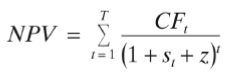where CFt = Capitalt + Interestt st is the zero coupon interest rate, derived from the appropriate swap curve, at valuation, to time t z is a static spread over the zero coupon interest rate, derived from the appropriate swap curve, that lets the net present value of the relevant exposure be equal to its price. 1.2 Floating interest rate assets The net present value of a relevant exposure, the interest payable on which is floating, shall be calculated as the discounted value of the principal or notional principal and interest outstanding from the next payment date, as follows: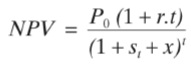where P0 is principal outstanding at the valuation date, time zero t time in years (or part of a year) to the next re-pricing date r is the annualised rate on the relevant exposure on a simple interest basis st the annualised Euribor or Libor zero-coupon rate, derived from the appropriate swap curve, to maturity t x a constant spread to the Euribor or Libor zero coupon rate, derived from the appropriate swap curve, that discounts the cash flows to par. 1.3 Fixed interest rate hedge contracts or commercial mortgage covered securities issued Subject to paragraph 1.6, the net present value of a hedge contract or commercial mortgage covered security issued, the interest payable on which is fixed, shall be calculated as the sum of the present values of each cashflow payable on the contract or security in each time period, as follows:where CFt = Interestt + Capitalt st is the zero coupon interest rate, derived from the appropriate swap curve, at valuation, to time t z is a static spread over the zero coupon interest rate, derived from the appropriate swap curve, that lets the net present value of the contract or security be equal to its price. 1.4 Floating interest rate hedge contracts or commercial mortgage covered securities issued Subject to paragraph 1.6, the net present value of a hedge contract or commercial mortgage covered security issued, the interest payable on which is floating, shall be calculated as the discounted value of the principal or notional principal and interest outstanding from the next payment date, as follows:where P0 is principal outstanding at the valuation date, time zero t time in years (or part of a year) to the next re-pricing date r is the annualised rate on the contract or security on a simple interest basis st is the zero coupon interest rate, derived from the appropriate swap curve, at valuation, to time t; and x is a static spread over the zero coupon interest rate, derived from the appropriate swap curve, that lets the net present value of the contract or security be equal to its price. 1.5 Total net present value of all relevant exposures The total net present value of all relevant exposures shall be calculated as the sum of the net present values of the relevant exposures. 1.6 Hedge contracts Where a hedge contract has a notional amount equal to the outstanding principal (or nominal) from time to time of commercial mortgage credit assets and/or substitution assets which are cover assets, or as applicable, not cover assets from time to time and that hedge contract has the hedging effect of converting a blended or averaged interest rate (determined by reference to various interest rates payable on those assets) into a single floating or fixed interest rate, that hedge contract shall be treated for the net present value purposes together with the related commercial mortgage credit assets and/or substitution assets as a single floating rate relevant exposure or, as applicable, single fixed rate relevant exposure. Where a hedge contract has a notional amount equal to the outstanding principal (or nominal) and term of one or more issues of commercial mortgage covered securities and that hedge contract has the hedging effect of converting the interest rate payable on those commercial mortgage covered securities into a single floating or fixed rate, that hedge contract shall be treated for net present value purposes together with the related commercial mortgage covered securities as a single floating rate asset or, as applicable, single fixed rate asset. The net present value of any other hedge contract shall be calculated, as appropriate, on the basis of the applicable formulae set out in paragraph 1.3 or 1.4. 2. Measurement of sensitivity to interest rate changes Designated commercial mortgage credit institutions shall use the following methodology in measuring their sensitivity to changes in interest rates. 2.1 Upward parallel shift in the yield curve For a one hundred basis points upward shift in the yield curve, the resultant net present value of relevant exposures shall be calculated as follows: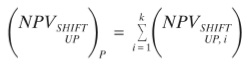where, for an individual relevant exposure, i: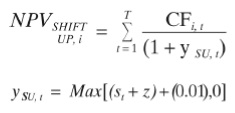st = zero coupon interest rate, derived from the appropriate swap curve, at valuation to time t; z is a static spread over the zero coupon interest rate, derived from the appropriate swap curve, that lets the net present value of the relevant exposure be equal to its price. 2.2 Downward parallel shift in the yield curve For a one hundred basis points downward shift in the yield curve, the resultant net present value of relevant exposures shall be calculated as: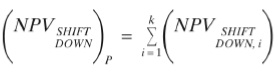where, for an individual relevant exposure, i: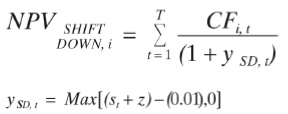st = zero coupon interest rate, derived from the appropriate swap curve, at valuation, to time t, z is the static spread over the zero coupon interest rate, derived from the appropriate swap curve, that lets the net present value of the relevant exposure be equal to its price. 2.3 Changes in the slope of the yield curve 2.3.1 General approach The procedure for the calculation of the net present value of relevant exposures resulting from a one hundred basis points twist in the yield curve shall be as follows: • for all revaluation points along the yield curve up to and including 3 months (i.e. the ‘short end’), increase (decrease) the yield curve values by 100 basis points; • for all revaluation points along the yield curve greater than or equal to 10 years (i.e. the ‘long end’), decrease (increase) the yield curve values by 100 basis points; and • in the interval from 3 months to 10 years, make proportional changes to the yield curve in accordance with the number of revaluation points in the interval. This new yield curve shall then be applied to all relevant exposures. The formulae for the calculation of the net present value of all relevant exposures resulting from a one hundred basis points twist in the yield curve are set out in paragraphs 2.3.2 and 2.3.3. 2.3.2 Downward change in the slope of the yield curve For a twist in the yield curve, up one hundred basis points in the short term and down one hundred basis points in the long term, with proportional changes in the interval, the resultant net present value of relevant exposures shall be calculated as: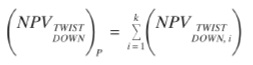where, for an individual relevant exposure, i: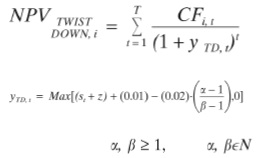α = 1 for t ≤ 3 months; α = β for t ≥ 10 years; for 3 months ≤ t ≤ 10 years, assign ascending values of α to each revaluation point along the curve greater than 3 months and less than 10 years, beginning with α = 1 for t = 3 months; β is the final revaluation point along the curve required in the interval (3 months ≤ t ≤ 10 years); z is a static spread over the zero coupon interest rate, derived from the appropriate swap curve, that lets the net present value of the relevant exposure be equal to its price. 2.3.3 Upward change in the slope of the yield curve For a twist in the yield curve, down one hundred basis points in the short term and up one hundred basis points in the long term, with proportional changes in the interval, the resultant net present value of relevant exposures shall be calculated as: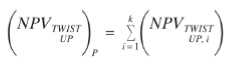where, for an individual relevant exposure, i: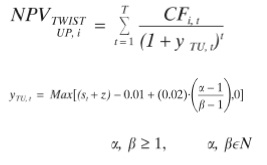α = 1 for t ≤ 3 months; α = β for t ≥ 10 years; for 3 months ≤ t ≤ 10 years, assign ascending values of α to each revaluation point along the curve greater than 3 months and less than 10 years, beginning with α = 1 for t = 3 months; β is the final revaluation point along the curve required in the interval (3 months ≤ t ≤ 10 years); z is a static spread over the zero coupon interest rate, derived from the appropriate swap curve, that lets the net present value of the relevant exposure be equal to its price. 2.4 Sensitivity to changes in interest rates Sensitivity to changes in interest rates shall be measured as the difference between: • actual net present value and the resultant net present value from a one hundred basis point upward shift in the yield curve; • actual net present value and the resultant net present value from a one hundred basis point downward shift in the yield curve; • actual net present value and the resultant net present value from a twist in the yield curve of plus one hundred basis points in the short term and minus one hundred basis points in the long term, with proportional changes in the interval; and • actual net present value and the resultant net present value from a twist in the yield curve of minus one hundred basis points in the short term and plus one hundred basis points in the long term, with proportional changes in the interval. 3. Reference interest rate curve The appropriate interest rate swap curve for each currency shall be used as the reference curve for valuing all relevant exposures.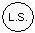Signed for and on behalf of THE IRISH FINANCIAL SERVICES REGULATORY AUTHORITY 17 June 2008 MR. PATRICK NEARY Chief Executive Irish Financial Services Regulatory Authority EXPLANATORY NOTE (This note is not part of the Instrument and does not purport to be a legal interpretation.) These Regulations prescribe formulae for calculation of sensitivity to interest rate changes for designated commercial mortgage credit institutions.